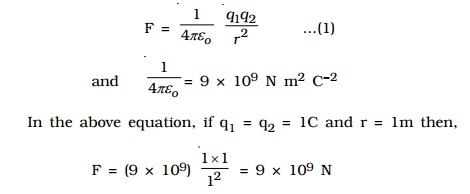Home | | Physics | Definition of Coulomb's law , Principle of Superposition, Electric Field, Electric Field Intensity (E)

# Definition of Coulomb's law , Principle of Superposition, Electric Field, Electric Field Intensity (E)

Coulomb's law The force between two charged bodies was studied by Coulomb in 1785. Coulomb's law states that the force of attraction or repulsion between two point charges is directly proportional to the product of the charges and inversely proportional to the square of the distance between them. The direction of forces is along the line joining the two point charges.

Coulomb's law

The force between two charged bodies was studied by Coulomb in 1785.

Coulomb's law states that the force of attraction or repulsion between two point charges is directly proportional to the product of the charges and inversely proportional to the square of the distance between them. The direction of forces is along the line joining the two point charges.

Let q1 and q2 be two point charges placed in air or vacuum at a distance r apart . Then, according to Coulomb's law,where k is a constant of proportionality. In air or vacuum,

k = 1/4??o , where ?o is the permittivity of free space (i.e., vacuum) and the value of ?o is 8.854 x 10?12 C2 N?1 m?2.One Coulomb is defined as the quantity of charge, which when placed at a distance of 1 metre in air or vacuum from an equal and similar charge, experiences a repulsive force of 9 x 109 N.

If the charges are situated in a medium of permittivity ?, then the magnitude of the force between them will be,Coulombxs law x vector formSo, the forces exerted by charges on each other are equal in magnitude and opposite in direction.

Principle of Superposition

The principle of superposition is to calculate the electric force experienced by a charge q1 due to other charges q2, q3 ..... qn.

The total force on a given charge is the vector sum of the forces exerted on it due to all other charges.Electric Field

Electric field due to a charge is the space around the test charge in which it experiences a force. The presence of an electric field around a charge cannot be detected unless another charge is brought towards it.

When a test charge qis placed near a charge q, which is the source of electric field, an electrostatic force F will act on the test charge.

Electric Field Intensity (E)

Electric field at a point is measured in terms of electric field intensity. Electric field intensity at a point, in an electric field is defined as the force experienced by a unit positive charge kept at that point.

It is a vector quantity.The unit of electric field intensity is N C?1

The electric field intensity is also referred as electric field strength or simply electric field. So, the force exerted by an electric field on a charge is F =  qoE.

Study Material, Lecturing Notes, Assignment, Reference, Wiki description explanation, brief detail
: Definition of Coulomb's law , Principle of Superposition, Electric Field, Electric Field Intensity (E) |

Related Topics